### 2.深度优先搜索(DFS)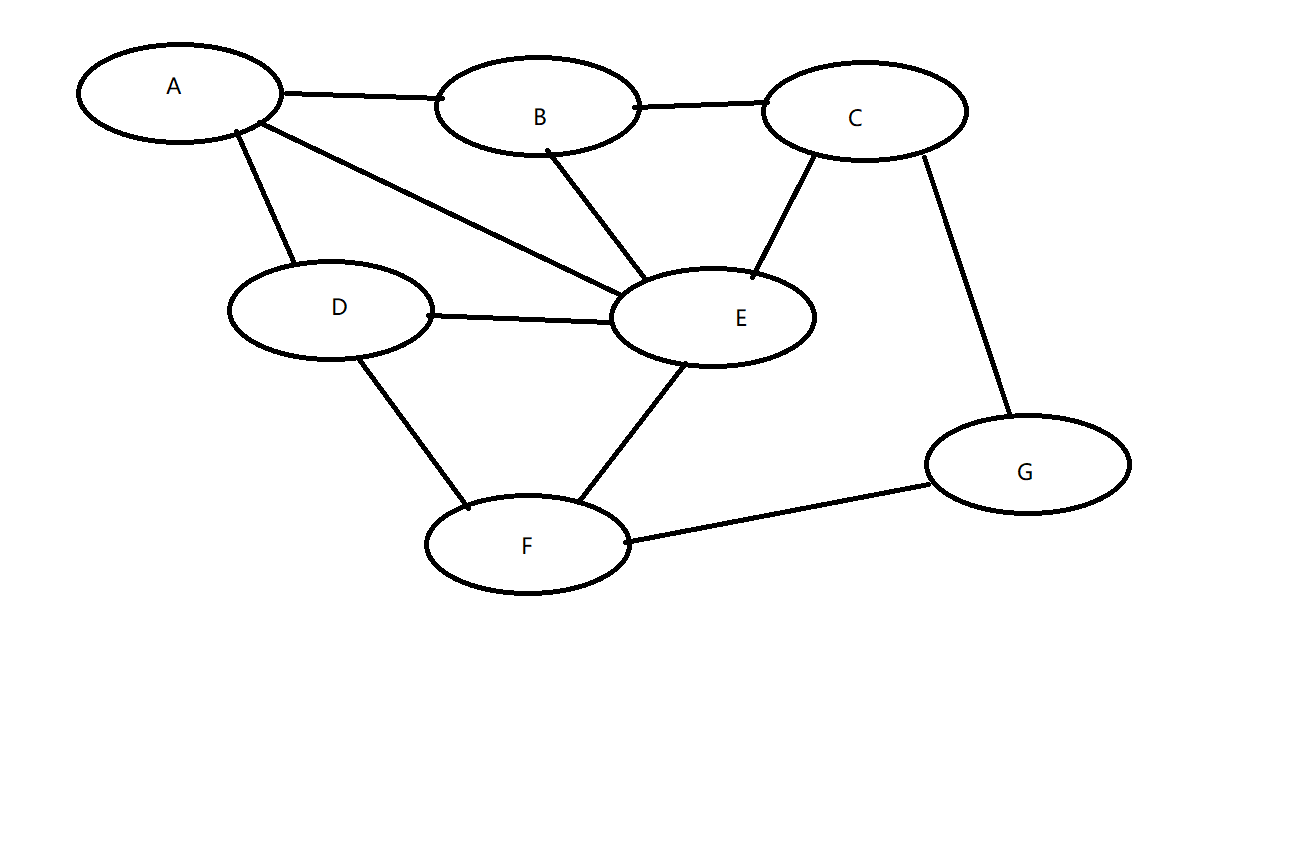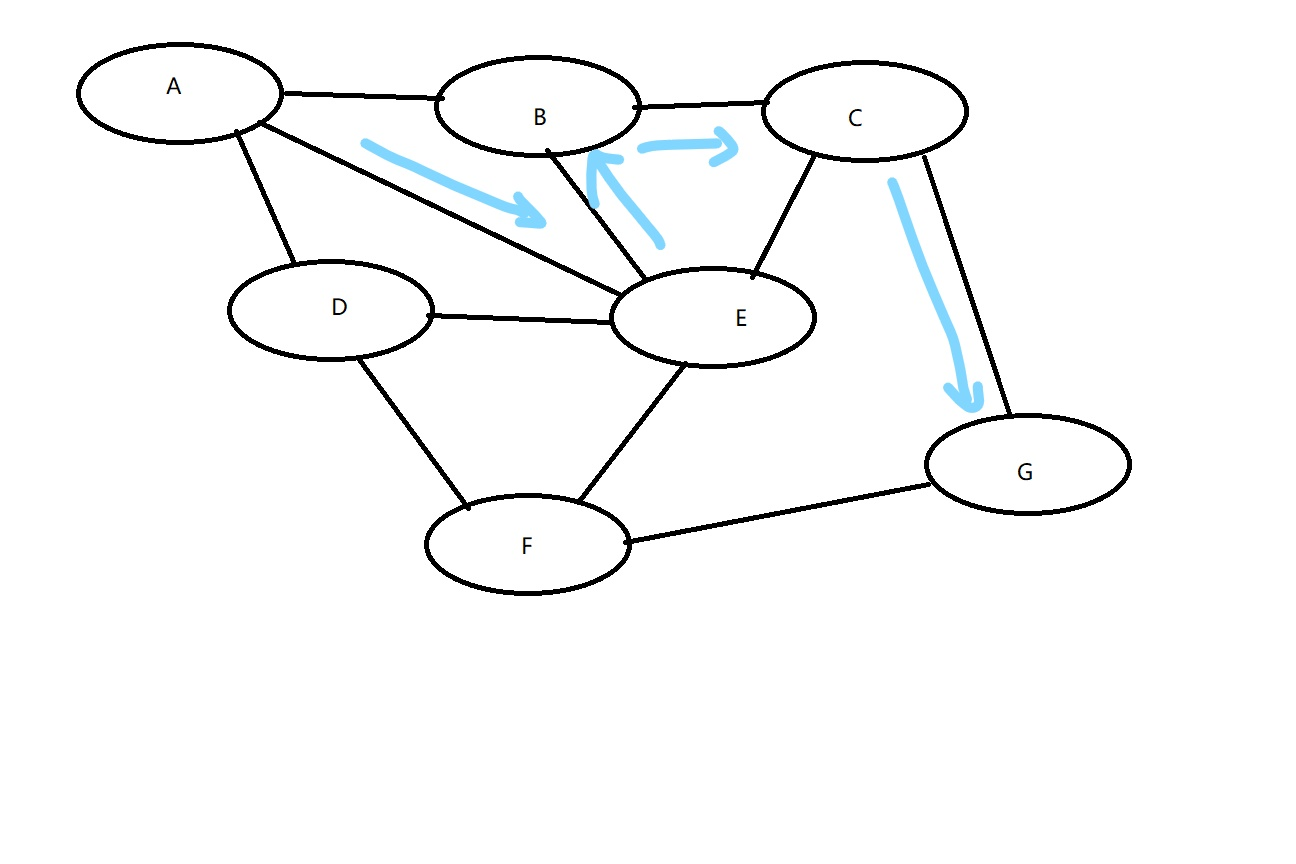1.把所有点标记为“未走过”；//把数组全标记为0或者其他

2.找到起点，终点并看看可以走到哪里；//准备循环

3.选择一节点并判断本节点是否走出地图；//

4.判断这一节点走过没啊；//3、4两步是判断走到该节点是否合法

5.如果没走过就走进该节点；//标记该节点

6.再寻找下一个节点；//深入下一层搜索

7.走到头了就可以回头了//得到返回这就可以回溯了

``````#include <iostream>
#include <cstdio>
using namespace std;
void dfs(/*起始坐标*/){
if(/*找到出口*/){
//操作
return ;
}
for(/*循环遍历所有方向*/){
if(/*新的坐标不符合条件*/)
continue;
//操作
dfs(/*继续向下搜索新的坐标*/);
//回溯
}
}
int main(){
//读入数据
dfs(/*起点坐标*/);

return 0;
}
``````

help庞学姐：

``````#include<stdio.h>
int n,m;
char mp;
int vis;
int dx[]={-1,1,0,0},dy[]={0,0,1,-1};
int ans=0,step=0,min=999999;
void dfs(int x,int y,int step){
if(x<0||x>=n||y<0||y>m) return ;
if(x==n-1&&y==m-1){ans=1;
if(step<min) min=step;
return ;}
for(int i=0;i<4;i++){
int nowx=x+dx[i];
int nowy=y+dy[i];
if(mp[nowx][nowy]=='*'&&vis[nowx][nowy]==0){
vis[nowx][nowy]=1;
dfs(nowx,nowy,step+1);
vis[nowx][nowy]=0;
}
}
}
int main(){
scanf("%d%d",&n,&m);
for(int i=0;i<n;i++)
scanf("%s",mp[i]);
dfs(0,0,0);
printf("%d",min);
return 0;
}
``````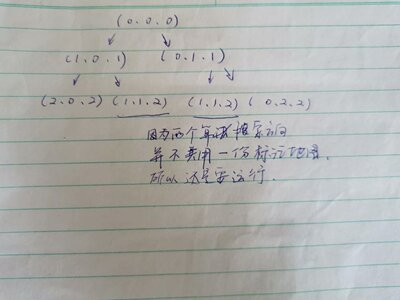### 3.广度优先搜索(BFS)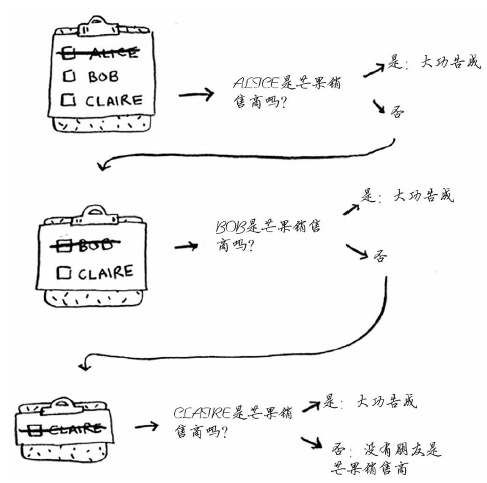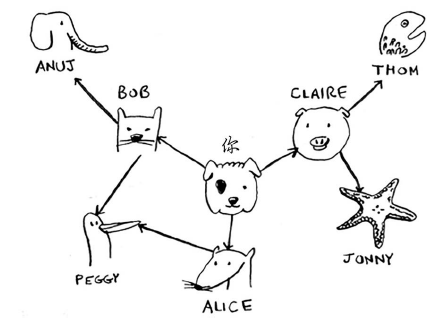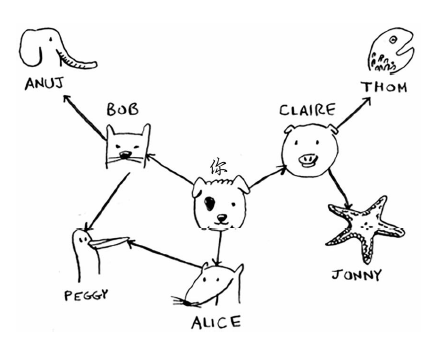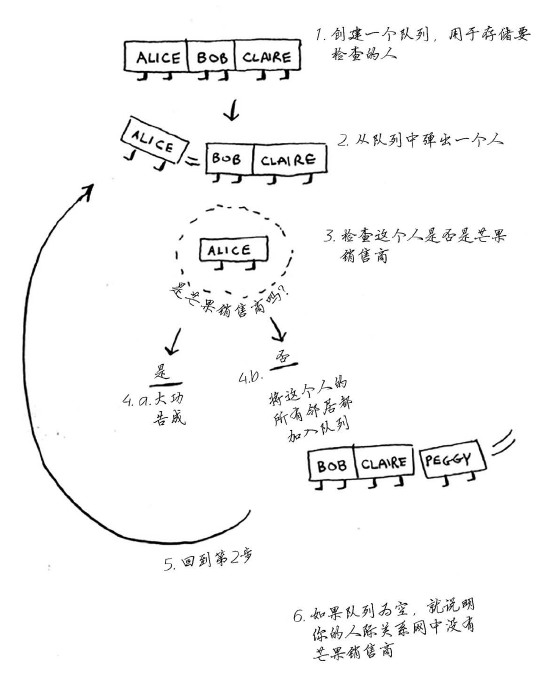``````#include<bits/stdc++.h>
using namespace std;
int n,m;
struct node {
int x,y,step;
node(int _a,int _b,int _c){x=_a,y=_b,step=_c;}
};
string mp;
int vis;
int dx[]={-1,1,0,0};
int dy[]={0,0,-1,1};
int bfs()
{
queue<node> q;
q.push(node(0,0,0));
while(!q.empty()){
node f=q.front();
q.pop();

for(int i=0;i<4;i++){
int nowx=f.x+dx[i];
int nowy=f.y+dy[i];

if(nowx<0||nowy<0||nowx>=n||nowy>=m)
continue;
if(nowx==n-1&&nowy==m-1)
return f.step+1;

if(mp[nowx][nowy]=='*'&&vis[nowx][nowy]==0)
{
q.push(node(nowx,nowy,f.step+1));
vis[nowx][nowy]=1;
}
}
}
}
int main()
{
cin>>n>>m;
for(int i=0;i<n;i++) cin>>mp[i];

cout<<bfs();

return 0;
}
``````